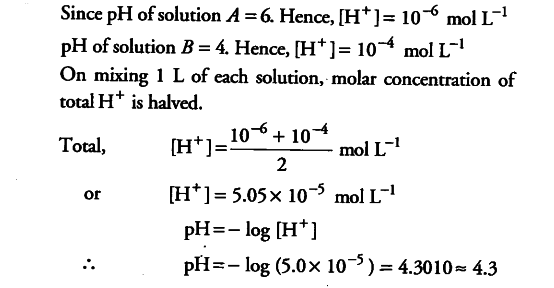# Calculate the pH of a solution formed by mixing equal volumes of two solutions,

Calculate the pH of a solution formed by mixing equal volumes of two solutions, A and B of a strong acid having pH = 6 and pH = 4 respectively.